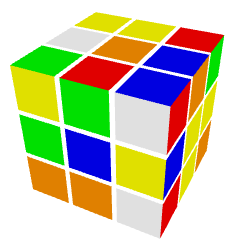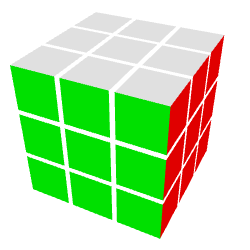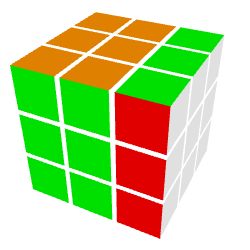# duksctf

## Bunch of sec. enthusiasts who sometimes play CTF

An interactive server implements a login challenge with a variant of Diffie-Hellman on the group of permutations of the Rubik’s cube. After computing a discrete logarithm on this (non-abelian) group, we can compute the handshake to log in as admin and obtain the flag.

### Description

We found some spies using Rubik’s cubes as paper weights. Can you break their crypto?

Challenge running at rubik.ctfcompetition.com:1337

### Details

Points: 298

Category: Cryptography

Validations: 20

### TL;DR

A solution in Python is available here, with a transcript of the communication. And here is an interactive visualization that takes as input cube configurations with the same serialization as in the challenge.Can you extract admin’s private key from this cube?

### Rust source code

We are given a source code in Rust that seems to implement a variant of Diffie-Hellman on the group of permutations of the Rubik’s cube. More precisely, given two generators $U$ and $L$, the public key associated to two private exponents $a$ and $b$ is the permutation $pk = L^b \circ U^a$. This public key is serialized as a configuration of the Rubik’s cube $pk(\textsf{default_cube})$, where $\textsf{default_cube}$ is a solved Rubik’s cube.

There is also some code to compute a handshake. Given a peer’s public key $pk$ and one’s private key $(a, b)$, the associated handshake is the permutation $L^b \circ pk \circ U^a$. Then again, this permutation is applied to the default cube, converted to a string and hashed (along with a salt) with BLAKE-2.

### Interactive server

The interactive server at rubik.ctfcompetition.com:1337 proposed several options.

\$ nc rubik.ctfcompetition.com 1337
Welcome to the Rubik's cube authentication server!

You have the following options:
1) Public key service
2) Register
q) Quit


Let’s start with option 1! We are prompted $a$ and $b$ and obtain a cube configuration.

1
What is your value of a?
0
What is your value of b?
0
Your public key is (0 * "U x'" + 0 * "L y'") ==
WWWWWWWWWGGGRRRBBBOOOGGGRRRBBBOOOGGGRRRBBBOOOYYYYYYYYY


Nice, so we can see that the default cube really looks like a solved cube, with colors {white, green, red, blue, orange, yellow}, serialized in the following order: top face, circles on the sides, bottom face.Rubik’s cube in default configuration

We can also extract $U$ and $L$ with $(a=1, b=0)$ and $(a=0, b=1)$.

U = OOOOOOGGGGGRWWWOBBYYYGGRWWWOBBYYYGGRWWWOBBYYYBBBRRRRRR
L = WWWWWWOOOOOYGGGWRRBBBOOYGGGWRRBBBOOYGGGWRRBBBRRRYYYYYYRubik’s cube after permutation URubik’s cube after permutation L

OK, now let’s try to register a new user. We are prompted for a public key, what to choose??

We are probably going to do a handshake with the server, so let’s try with a zero key, i.e. $(a=0, b=0)$ and $pk = \textsf{default_cube}$. That way, the handshake computed by a peer will collapse to the peer’s public key, no need to extract their secret key.

2
What username do you want to register?
user
What public key do you want to register?
WWWWWWWWWGGGRRRBBBOOOGGGRRRBBBOOOGGGRRRBBBOOOYYYYYYYYY
User registered!


We can now log in. We are challenged to compute the handshake with the server’s public key.

3
user
My public key is:
WYBORYGWYBWWOBRGGRYRRRWOYGRGYGOBBOYGYORGRBOGWOBWBOWBWY

Please give me the result of:
mykey.handshake(yourkey, "420823cb18a31058".from_hex().unwrap()).to_hex()


As expected, we are challenged to obtain the handshake that a peer would compute with their secret key and our public key. Since we chose a dummy public key, the handshake’s cube is equal to the peer’s public key! The same would happen in any Diffie-Hellman handshake if someone selects a zero exponent.An example of challenger’s public key

The server quickly closes the connection, so let’s automate the computation. I used Python2 with pyblake2 library for the hash. However, after some failed attempts and looking at Rust’s BLAKE-2 code, it turns out that what the CTF calls salt is what BLAKE-2 calls key!

sock = socket.socket()
# ...
h = blake2b(key=salt, digest_size=16)
h.update(cube)
handshake = h.hexdigest()
sock.send(handshake + '\n')


We are now logged in! And the flag is…

Not here.

However, we have a new option to list users.

4) List users


We obtain a list of logins and public keys.

Username: admin
Key: GWYROYBBRYGWROGYGBRROGBYBYYRGBWWWOOWRYOWRYBGWGOGWRBBOO
Key: WWWWWWWWWGGGRRRBBBOOOGGGRRRBBBOOOGGGRRRBBBOOOYYYYYYYYY


At this point we cannot use the zero private key trick anymore to compute admin’s handshake. We really need to extract secret keys from public keys.

### Finding the generator permutations $U$ and $L$

Given a public key $\textsf{cube}$, we could try to bruteforce $a$ and $b$ and check whether $\textsf{cube} = L^b \circ U^a (\textsf{default_cube})$. However, we need to implement arithmetic on Rubik’s cubes. I have already played with a Rubik’s cube before and $L/U$ probably means turn the left/up face one quarter round, but it’s not obvious to visualize from those long strings of 6 letters…

This is when I realized that permutations on the cube can just be seen as permutations of 54 letters! So for $U$ we just need to find a permutation of $\{1, \ldots, 54\}$ such that WWWWWWWWWGGGRRRBBBOOOGGGRRRBBBOOOGGGRRRBBBOOOYYYYYYYYY is mapped to OOOOOOGGGGGRWWWOBBYYYGGRWWWOBBYYYGGRWWWOBBYYYBBBRRRRRR.

Unfortunately, there are only 6 colors so there are many possible permutations, e.g. the first W can be mapped to 9 locations. To retrieve the exact permutation, I simply asked the server $U^a(\textsf{default_cube})$ for $a = 0, 1, \ldots$ and derive constraints on $U$ by comparing pairs $(U^a(\textsf{default_cube}), U^{a+1}(\textsf{default_cube}))$.

# Solve permutation U
def solveU(sock):
cube = getcubeU(sock, 0)
# Init a NxN matrix with ones
constraints = []
for i in range(N):
constraints.append( * N)

k = 0
while True:
k += 1
newcube = getcubeU(sock, k)

# Update constraints on permutation
for i in range(N):
for j in range(N):
if cube[i] != newcube[j]:
constraints[i][j] = 0
cube = newcube

# Try to extract the permutation
p = getperm(constraints)
if p is not None:
return p

##### Util functions #####

# Extract a permutation from constraints, or None if several solutions exist
def getperm(constraints):
l =  * N
for i in range(N):
found = False
for j in range(N):
if constraints[i][j] == 1:
if found:
return None
found = True
l[i] = j
return l

# Read line from a socket
def recvline(sock):
buf = ''
while True:
tmp = s.recv(1)
if len(tmp) == 0:
raise socket.error
buf += tmp
if tmp == '\n':
break
return buf

# Read a cube for (a, b=0)
def getcubeU(sock, a):
for i in range(6):
recvline(sock)

sock.send(sock, '1' + '\n')
recvline(sock)
sock.send(str(a) + '\n')
recvline(sock)
sock.send(str(b) + '\n')
recvline(sock)
cube = recvline(sock)
return cube


After 12 iterations, the set of constraints reduces to only one possible permutation (same for $L$). Here are $U$ and $L$ (using the convention that $i$ is mapped to the value of cell $U[i]$).

U = [14, 26, 38, 13, 25, 37, 12, 24, 36,
8,  7,  6, 11, 23, 35, 45, 46, 47,
39, 27, 15, 10, 22, 34, 48, 49, 50,
40, 28, 16,  5,  4,  3,  9, 21, 33,
51, 52, 53, 41, 29, 17,  2,  1,  0,
44, 43, 42, 32, 31, 30, 20, 19, 18]
L = [15,  3,  0, 27,  4,  1, 39,  5,  2,
14, 26, 38, 47, 16, 17, 18, 19, 20,
9, 10,  8, 13, 25, 37, 46, 28, 29,
30, 31, 32, 21, 22,  7, 12, 24, 36,
45, 40, 41, 42, 43, 44, 33, 34,  6,
35, 50, 53, 23, 49, 52, 11, 48, 51]


### Discrete logarithm in subgroup of Rubik’s cube permutations

A quick check shows that $U$ and $L$ both have order 1260, which is fairly small! We could brute-force all pairs $(a, b)$ for $0 \le a, b < 1260$, but a more efficient method is to adapt the baby-step giant-step algorithm to our (non-abelian) group of permutations.

Namely, we start by caching in a hash table the pairs $(U^a(\textsf{default_cube}), a)$ for $0 \le a < 1260$. Then, given a public key $\textsf{cube}$ to break, we compute $L^{-b}(\textsf{cube})$ for $0 \le b < 1260$ until there is a match $L^{-b}(\textsf{cube}) = U^a(\textsf{default_cube})$ in the hash table. The corresponding pair $(a, b)$ is the secret key.

I applied this discrete logarithm algorithm to both the challenger’s public key and the admin’s public key, hoping to find two pairs $(a, b)$ and $(a', b')$ and obtaining the handshake as $L^{b+b'} \circ U^{a+a'} (\textsf{default_cube})$.

However, only the challenger’s public key factorizes! It seems that the admin cheated and did not provide a public key of the form $L^b \circ U^a$…

### Extracting a permutation between two cubes

OK so we are back to the following problem: given a cube configuration, what is the permutation $P$ of its 54 squares such that $\textsf{cube} = P(\textsf{default_cube})$? The 6 colors are repeating, so this time we really need to visualize a Rubik’s cube…

It turns out that the physical structure of the cube gives more constraints: corners can only be permuted with corners, sides can only be permuted with sides, and centers can only be permuted with centers. What’s more, the 3 squares that form a corner are bound together, and same goes for the 2 squares that form a side. Finally, given 3 colors (e.g. white, red, blue), there is at most one corner that combines these colors. Same goes for sides.

Great, so now we have to identify where centers/sides/corners are on the 54-character strings… The default cube has clear patterns and if we assume that it is a solved cube we can already guess that squares are numbered in the following order: top face, circles on the sides, bottom face. By looking more closely, permutation $U$ seems to rotate the top face by a quarter round, then rotate the whole cube. This gives some insight about the order of traversal of the top and bottom faces, and after some manual testing I ended up finding a mapping between $\{1, \ldots, 54\}$ and the squares on the physical cube.

Once the correct mapping is found, we can extract a permutation between two cubes!

# Extract permutation between the default cube and any cube
def cube2perm(zero, cube):
# Indices of distinguished squares
CENTERS = [
4, # top
22, 25, 28, 31, # sides
49 # bottom
]
SIDES = [
(3,10), (7,13), (5,16), (1,19), # top
(23,24), (26,27), (29,30), (32,21), # sides
(34,48), (37,46), (40,50), (43,52) # bottom
]
CORNERS = [
(0,9,20), (6,11,12), (8,14,15), (2,17,18), # top
(51,44,33), (45,35,36), (47,38,39), (53,41,42) # bottom
]

perm =  * N

for i in CENTERS:
for a in CENTERS:
if zero[i] == cube[a]:
perm[i] = a
break

for i, j in SIDES:
for a, b in SIDES:
if sorted([zero[i], zero[j]]) == sorted([cube[a], cube[b]]):
if zero[i] == cube[b]:
a, b = b, a
perm[i] = a
perm[j] = b
break

for i, j, k in CORNERS:
for a, b, c in CORNERS:
if sorted([zero[i], zero[j], zero[k]]) == sorted([cube[a], cube[b], cube[c]]):
if zero[i] == cube[b]:
a, b = b, a
elif zero[i] == cube[c]:
a, c = c, a
if zero[j] == cube[c]:
b, c = c, b
perm[i] = a
perm[j] = b
perm[k] = c
break

return perm


### Wrapping-up

So now we can put everything together to log in as admin and extract the flag! First, we extract admin’s permutation $P_{admin}$ from its public key using the above cube2perm function. Then, when challenged to find a handshake from some public key $pk$, we extract the associated $a$ and $b$ as $pk = L^b \circ U^a(\textsf{default_cube})$ and we can compute the handshake $L^b \circ P_{admin} \circ U^a(\textsf{default_cube})$ to log in.

Here is a Python script that solves the challenge: rubik.py. I also recorded a transcript of the communication.

The flag is CTF{StickelsKeyExchangeByHand}.

You can also play with my interactive visualization of cube configurations in webGL here.

First, can both peers compute the same handshake? If everyone uses a public key of the form $L^b \circ U^a$, the handshake is indeed symmetric:
$L^b \circ (L^{b'} \circ U^{a'}) \circ U^a = L^{b'} \circ (L^b \circ U^a) \circ U^{a'}$